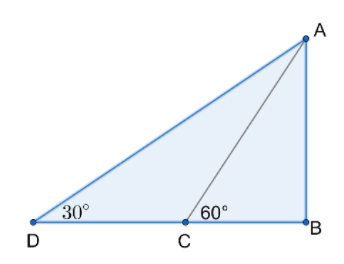Courses
Courses for Kids
Free study material
Free LIVE classes
MoreLIVE
Join Vedantu’s FREE Mastercalss

# The shadow of a tower standing on level ground is found to be $40m$ longer when the sun's altitude is $30{}^\circ$ than when it is $60{}^\circ$. Find the height of the tower.Verified
259.5k+ views
Hint: First draw a figure so that you can understand. Label the figure according to the question. Consider one Right-angled triangle and take the $\tan 60{}^\circ$ and for the next Right-angled triangle take $\tan 30{}^\circ$. Equate it and find the height.

Height is a measure of vertical distance, either vertical extent (how "tall" something or someone is) or vertical position (how "high" a point is). For example, "The height of that building is $50m$" or "The height of an airplane in-flight is about $10000m$".
When the term is used to describe the vertical position (of, e.g., an airplane) from sea level, height is more often called altitude. Furthermore, if the point is attached to the Earth (e.g., a mountain peak), the altitude (height above sea level) is called elevation.
Height may indicate the third dimension, the other two being length and width. Height is normal to the plane formed by the length and width.
Height is also used as a name for some more abstract definitions. These include:
The altitude of a triangle, which is the length from the vertex of a triangle to the line formed by the opposite side.
Measurement in a circular segment of the distance from the midpoint of the arc of the circular segment to the midpoint of the line joining the endpoints of the arc.
In a rooted tree, the height of a vertex is the length of the longest downward path to a leaf from that vertex.
In algebraic number theory, a "height function" is a measurement related to the minimal polynomial of an algebraic number; among other uses in commutative algebra and representation theory.
In-ring theory, the height of a prime ideal is the supremum of the lengths of all chains of prime ideals contained in it.Let the tower be $AB$.
When the sun’s altitude is at $60{}^\circ$, $\angle ACB=60{}^\circ$and the length of the shadow$=BC$.
When the sun’s altitude is at $30{}^\circ$, $\angle ADB=30{}^\circ$and the length of the shadow$=DB$.
So the length of the shadow is $40m$ when the angle changes from $60{}^\circ$ to $30{}^\circ$.
That is $CD=40m$.
Now we have to find the height of the tower i.e. $AB$.
Here we can see that tower is vertical to ground so $\angle ABC=90{}^\circ$.
So considering Right-angled triangle $ABC$,
$\tan C=\dfrac{Side\text{ }opposite\text{ }to\text{ }angleC}{Side\text{ }adjacent\text{ }to\text{ }angleC}=\dfrac{AB}{CB}$
$\tan 60{}^\circ =\dfrac{AB}{CB}$
$\sqrt{3}=\dfrac{AB}{CB}$

$CB=\dfrac{AB}{\sqrt{3}}$ ………… (1)
Now considering Right-angled triangle $ADC$,
$\tan D=\dfrac{Side\text{ }opposite\text{ }to\text{ }angleD}{Side\text{ }adjacent\text{ }to\text{ }angleD}=\dfrac{AB}{DB}$
$\tan 30{}^\circ =\dfrac{AB}{DB}$
$\dfrac{1}{\sqrt{3}}=\dfrac{AB}{DB}$
$DB=\sqrt{3}AB$
So we know $DC+CB=DB$.
$40+CB=\sqrt{3}AB$
$CB=\sqrt{3}AB-40$ ………. (2)
From (1) and (2),
$\dfrac{AB}{\sqrt{3}}=\sqrt{3}AB-40$
$AB=\sqrt{3}\times \sqrt{3}AB-40\sqrt{3}$
$AB=3AB-40\sqrt{3}$
$40\sqrt{3}=3AB-AB$
$2AB=40\sqrt{3}$
$AB=20\sqrt{3}m$
So here we get the height of the tower $AB=20\sqrt{3}m$.

Note: Carefully read the question. Your concept regarding height should be cleared. You should also know the substitution. Also, you must know to draw a figure first so you can find the answer quickly. Most mistakes are done by students at $\tan 60{}^\circ$and$\tan 30{}^\circ$. The students are confused between these two.
Last updated date: 17th Sep 2023
Total views: 259.5k
Views today: 3.59k